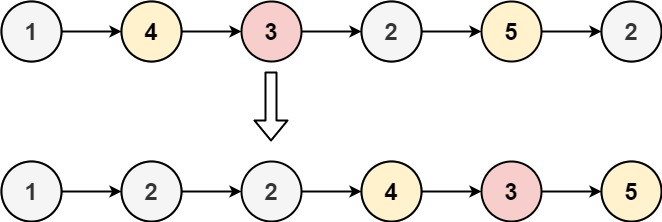304 North Cardinal St.
Dorchester Center, MA 02124

# Partition List LeetCode Solution

## Problem – Partition List LeetCode Solution

Given the `head` of a linked list and a value `x`, partition it such that all nodes less than `x` come before nodes greater than or equal to `x`.

You should preserve the original relative order of the nodes in each of the two partitions.

Example 1:``````Input: head = [1,4,3,2,5,2], x = 3
Output: [1,2,2,4,3,5]
``````

Example 2:

``````Input: head = [2,1], x = 2
Output: [1,2]
``````

Constraints:

• The number of nodes in the list is in the range `[0, 200]`.
• `-100 <= Node.val <= 100`
• `-200 <= x <= 200`

## Partition List LeetCode Solution in Python

``````class Solution:
def partition(self, head: ListNode, x: int) -> ListNode:
fdum, bdum = ListNode(0), ListNode(0)
front, back, curr = fdum, bdum, head
while curr:
if curr.val < x:
front.next = curr
front = curr
else:
back.next = curr
back = curr
curr = curr.next
front.next, back.next = bdum.next, None
return fdum.next
``````

## Partition List LeetCode Solution in Java

``````class Solution {
public ListNode partition(ListNode head, int x) {
ListNode fdum = new ListNode(0), bdum = new ListNode(0),
front = fdum, back = bdum, curr = head;
while (curr != null) {
if (curr.val < x) {
front.next = curr;
front = curr;
} else {
back.next = curr;
back = curr;
}
curr = curr.next;
}
front.next = bdum.next;
back.next = null;
return fdum.next;
}
}
``````

## Partition List LeetCode Solution in C++

``````class Solution {
public:
ListNode* partition(ListNode* head, int x) {
ListNode *fdum = new ListNode(0), *bdum = new ListNode(0),
*front = fdum, *back = bdum, *curr = head;
while (curr) {
if (curr->val < x) front->next = curr, front = curr;
else back->next = curr, back = curr;
curr = curr->next;
}
front->next = bdum->next, back->next = nullptr;
return fdum->next;
}
};
``````
##### Partition List LeetCode Solution Review:

In our experience, we suggest you solve this Partition List LeetCode Solution and gain some new skills from Professionals completely free and we assure you will be worth it.

If you are stuck anywhere between any coding problem, just visit Queslers to get the Partition List LeetCode Solution

Find on Leetcode

##### Conclusion:

I hope this Partition List LeetCode Solution would be useful for you to learn something new from this problem. If it helped you then don’t forget to bookmark our site for more Coding Solutions.

This Problem is intended for audiences of all experiences who are interested in learning about Data Science in a business context; there are no prerequisites.

Keep Learning!

More Coding Solutions >>

LeetCode Solutions

Hacker Rank Solutions

CodeChef Solutions